Home Practice
For learners and parents For teachers and schools
Textbooks
Full catalogue
Pricing SupportLog in

We think you are located in United States. Is this correct?

# 4.2 Equation of a line

## 4.2 Equation of a line (EMBG8)

We can derive different forms of the straight line equation. The different forms are used depending on the information provided in the problem:

• The two-point form of the straight line equation: $$\dfrac{y-y_1}{x-x_1} = \dfrac{y_2-y_1}{x_2-x_1}$$
• The gradient–point form of the straight line equation: $$y-y_1 = m(x-x_1)$$
• The gradient–intercept form of the straight line equation: $$y = mx + c$$

### The two-point form of the straight line equation (EMBG9)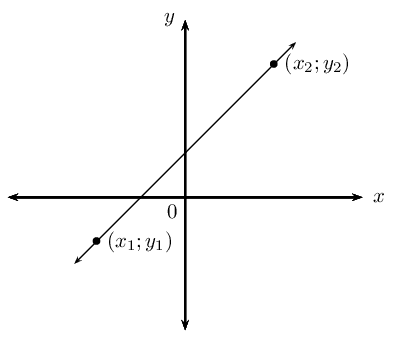Given any two points $$\left({x}_{1};{y}_{1}\right)$$ and $$\left({x}_{2};{y}_{2}\right)$$, we can determine the equation of the line passing through the two points using the equation:

$\frac{y-y_1}{x-x_1} = \frac{y_2-y_1}{x_2-x_1}$

## Worked example 3: The two-point form of the straight line equation

Find the equation of the straight line passing through $$P\left(-1;-5\right)$$ and $$Q\left(5;4\right)$$.

### Draw a sketch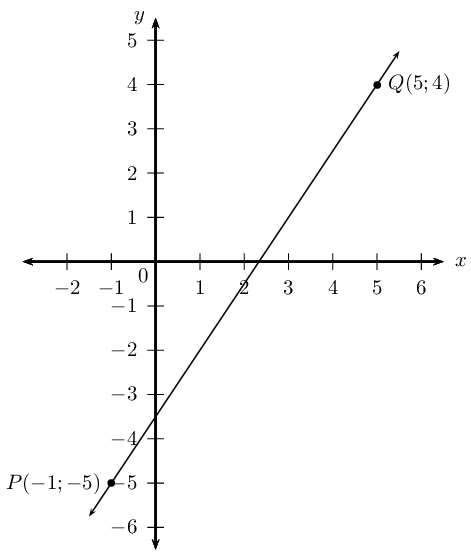### Assign variables to the coordinates of the given points

Let the coordinates of $$P$$ be $$(x_1;y_1)$$ and $$Q(x_2;y_2)$$

$x_1 = -1; \qquad y_1 = -5; \qquad x_2 = 5; \qquad y_2 = 4$

### Write down the two-point form of the straight line equation

$\frac{y-y_1}{x-x_1} = \frac{y_2-y_1}{x_2-x_1}$

### Substitute the values and make $$y$$ the subject of the equation

\begin{align*} \frac{y-(-5)}{x-(-1)} &= \frac{4-(-5)}{5-(-1)} \\ \frac{y+5}{x+1} &= \frac{9}{6} \\ y+5 &= \frac{3}{2}(x+1) \\ y+5 &= \frac{3}{2}x + \frac{3}{2} \\ y &= \frac{3}{2}x - \frac{7}{2} \end{align*}

$y = \frac{3}{2}x - 3\frac{1}{2}$

## The two-point form of the straight line equation

Textbook Exercise 4.2

Determine the equation of the straight line passing through the points:

$$(3;7)$$ and $$(-6;1)$$

\begin{align*} \frac{y-y_1}{x-x_1} &= \frac{y_2-y_1}{x_2-x_1} \\ \frac{y-7}{x-3} &= \frac{1-7}{-6-3} \\ \frac{y-7}{x-3} &= \frac{-6}{-9} \\ \frac{y-7}{x-3} &= \frac{2}{3} \\ y - 7 &= \frac{2}{3} \left( x - 3 \right) \\ y &= \frac{2}{3}x - 2 + 7 \\ \therefore y &= \frac{2}{3}x + 5 \end{align*}

$$(1;-\frac{11}{4})$$ and $$(\frac{2}{3}; -\frac{7}{4})$$

\begin{align*} \frac{y-y_1}{x-x_1} &= \frac{y_2-y_1}{x_2-x_1} \\ \frac{y + \frac{11}{4}}{x-1} &= \frac{-\frac{7}{4} + \frac{11}{4}}{\frac{2}{3} - 1} \\ \frac{y + \frac{11}{4}}{x-1} &= \frac{1}{-\frac{1}{3}} \\ \frac{y + \frac{11}{4}}{x-1} &= -3 \\ y + \frac{11}{4} &= -3 (x - 1) \\ y &= -3x + 3 - \frac{11}{4} \\ \therefore y &= -3x + \frac{1}{4} \end{align*}

$$(-2;1)$$ and $$(3;6)$$

\begin{align*} \frac{y-y_1}{x-x_1} &= \frac{y_2-y_1}{x_2-x_1} \\ \frac{y-1}{x+2} &= \frac{6-1}{3+2} \\ \frac{y-1}{x+2} &= \frac{5}{5} \\ y - 1 &= x + 2 \\ \therefore y &= x + 3 \end{align*}

$$(2;3)$$ and $$(3;5)$$

\begin{align*} \frac{y-y_1}{x-x_1} &= \frac{y_2-y_1}{x_2-x_1} \\ \frac{y-3}{x-2} &= \frac{5-3}{3-2} \\ \frac{y-3}{x-2} &= 2 \\ y - 3 &= 2(x - 2) \\ y &= 2x - 4 + 3\\ \therefore y &= 2x - 1 \end{align*}

$$(1;-5)$$ and $$(-7;-5)$$

\begin{align*} \frac{y-y_1}{x-x_1} &= \frac{y_2-y_1}{x_2-x_1} \\ \frac{y+5}{x-1} &= \frac{-5+5}{-7-1} \\ \frac{y+5}{x-1} &= 0 \\ y + 5 &= 0\\ \therefore y &= -5 \end{align*}

$$(-4;0)$$ and $$(1;\frac{15}{4})$$

\begin{align*} \frac{y-y_1}{x-x_1} &= \frac{y_2-y_1}{x_2-x_1} \\ \frac{y-0}{x+4} &= \frac{\frac{15}{4}-0}{1+4} \\ \frac{y}{x+4} &= \frac{\frac{15}{4}}{5} \\ \frac{y}{x+4} &= \frac{15}{20} \\ y &= \frac{3}{4} \left( x+4 \right) \\ \therefore y &= \frac{3}{4}x + 3 \end{align*}

$$(s;t)$$ and $$(t;s)$$

\begin{align*} \frac{y-y_1}{x-x_1} &= \frac{y_2-y_1}{x_2-x_1} \\ \frac{y-t}{x-s} &= \frac{s - t}{t - s}\\ \frac{y-t}{x-s} &= \frac{-(t - s)}{t - s}\\ \frac{y-t}{x-s} &= -1\\ y - t &= -(x - s)\\ y &= -x + s + t \end{align*}

$$(-2;-8)$$ and $$(1;7)$$

\begin{align*} \frac{y-y_1}{x-x_1} &= \frac{y_2-y_1}{x_2-x_1} \\ \frac{y+8}{x+2} &= \frac{7+8}{1+2} \\ \frac{y+8}{x+2} &= \frac{15}{3} \\ \frac{y+8}{x+2} &= 5 \\ y + 8 &= 5(x + 2)\\ y + 8 &= 5x + 10\\ y &= 5x + 2 \end{align*}

$$(2p;q)$$ and $$(0;-q)$$

\begin{align*} \frac{y-y_1}{x-x_1} &= \frac{y_2-y_1}{x_2-x_1} \\ \frac{y-q}{x-2p} &= \frac{-q-q}{0 - 2p} \\ \frac{y-q}{x-2p} &= \frac{-2q}{-2p} \\ \frac{y-q}{x-2p} &= \frac{q}{p} \\ y - q &= \frac{q}{p} \left( x - 2p \right) \\ y - q &= \frac{q}{p}x - 2q \\ \therefore y &= \frac{q}{p}x - q \end{align*}

### The gradient–point form of the straight line equation (EMBGB)

We derive the gradient–point form of the straight line equation using the definition of gradient and the two-point form of a straight line equation $\frac{y-y_1}{x-x_1} = \frac{y_2-y_1}{x_2-x_1}$

Substitute $$m = \dfrac{y_2-y_1}{x_2-x_1}$$ on the right-hand side of the equation $\frac{y-y_1}{x-x_1} = m$

Multiply both sides of the equation by $$(x-x_1)$$ $y-y_1 = m(x-x_1)$

To use this equation, we need to know the gradient of the line and the coordinates of one point on the line.

## Worked example 4: The gradient–point form of the straight line equation

Determine the equation of the straight line with gradient $$m = -\frac{1}{3}$$ and passing through the point $$(-1;1)$$.

### Draw a sketch

We notice that $$m < 0$$, therefore the graph decreases as $$x$$ increases.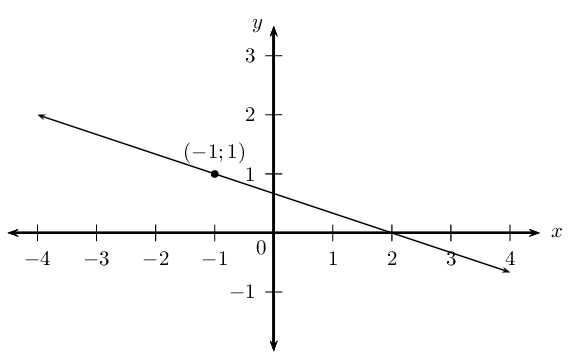### Write down the gradient–point form of the straight line equation

$y - y_1= m(x - x_1)$

Substitute the value of the gradient

$y - y_1= -\frac{1}{3}(x - x_1)$

Substitute the coordinates of the given point

\begin{align*} y - 1 &= -\frac{1}{3}(x - (-1)) \\ y - 1 &= -\frac{1}{3}(x + 1) \\ y &= -\frac{1}{3}x - \frac{1}{3} + 1\\ &= -\frac{1}{3}x + \frac{2}{3} \end{align*}

The equation of the straight line is $$y = -\frac{1}{3}x +\frac{2}{3}$$.

If we are given two points on a straight line, we can also use the gradient–point form to determine the equation of a straight line. We first calculate the gradient using the two given points and then substitute either of the two points into the gradient–point form of the equation.

## Worked example 5: The gradient–point form of the straight line equation

Determine the equation of the straight line passing through $$\left(-3;2\right)$$ and $$\left(5;8\right)$$.

### Draw a sketch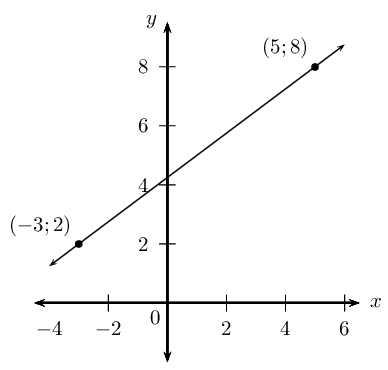### Assign variables to the coordinates of the given points

$x_1 = -3; \qquad y_1 = 2; \qquad x_2 = 5; \qquad y_2 = 8$

### Calculate the gradient using the two given points

\begin{align*} m &= \frac{y_2 - y_1}{x_2 - x_1} \\ &= \frac{8 - 2}{5 - (- 3)} \\ &= \frac{6}{8} \\ &= \frac{3}{4} \end{align*}

### Write down the gradient–point form of the straight line equation

$y - y_1= m(x - x_1)$

Substitute the value of the gradient

$y - y_1= \frac{3}{4}(x - x_1)$

Substitute the coordinates of a given point

\begin{align*} y - y_1 &= \frac{3}{4}(x - x_1) \\ y - 2 &= \frac{3}{4}(x - (-3)) \\ y - 2 &= \frac{3}{4}(x + 3) \\ y &= \frac{3}{4}x + \frac{9}{4} + 2\\ &= \frac{3}{4}x + \frac{17}{4} \end{align*}

The equation of the straight line is $$y = \frac{3}{4}x +4\frac{1}{4}$$.

## Gradient–point form of a straight line equation

Textbook Exercise 4.3

Determine the equation of the straight line:

passing through the point $$(-1;\frac{10}{3})$$ and with $$m=\frac{2}{3}$$.

\begin{align*} y - y_1 &= m(x - x_1)\\ y - \frac{10}{3} &= \frac{2}{3}(x + 1)\\ y - \frac{10}{3} &= \frac{2}{3}x + \frac{2}{3} \\ y &= \frac{2}{3}x + \frac{2}{3} + \frac{10}{3} \\ \therefore y &= \frac{2}{3}x + 4 \end{align*}

with $$m= -1$$ and passing through the point $$(-2;0)$$.

\begin{align*} y - y_1 &= m(x - x_1) \\ y - 0 &= -(x + 2) \\ \therefore y &= -x - 2 \end{align*}

passing through the point $$(3;-1)$$ and with $$m=-\frac{1}{3}$$.

\begin{align*} y - y_1 &= m(x - x_1) \\ y + 1 &= -\frac{1}{3}(x - 3) \\ y + 1 &= -\frac{1}{3}x + 1 \\ \therefore y &= -\frac{1}{3}x \end{align*}

parallel to the $$x$$-axis and passing through the point $$(0;11)$$.

\begin{align*} y - y_1 &= m(x - x_1) \\ y - 11 &= 0(x - 0) \\ \therefore y &= 11 \end{align*}

passing through the point $$(1;5)$$ and with $$m=-2$$.

\begin{align*} y - y_1 &= m(x - x_1) \\ y - 5 &= -2(x - 1) \\ y - 5 &=-2x + 2 \\ \therefore y &= -2x + 7 \end{align*}

perpendicular to the $$x$$-axis and passing through the point $$(-\frac{3}{2};0)$$.

$$x = -\frac{3}{2}$$

with $$m = -\text{0,8}$$ and passing through the point $$(10;-7)$$.

\begin{align*} y - y_1 &= m(x - x_1) \\ y + 7 &= -\frac{4}{5}(x - 10) \\ y + 7 &= -\frac{4}{5}x + 8 \\ \therefore y &= -\frac{4}{5}x + 1 \end{align*}

with undefined gradient and passing through the point $$(4;0)$$.

$$x = 4$$

with $$m= 3a$$ and passing through the point $$(-2;-6a+b)$$.

\begin{align*} y - y_1 &= m(x - x_1) \\ y - (-6a + b) &= 3a(x + 2) \\ y + 6a - b &= 3ax + 6a \\ \therefore y &= 3ax + b \end{align*}

### The gradient–intercept form of a straight line equation (EMBGC)

Using the gradient–point form, we can also derive the gradient–intercept form of the straight line equation.

Starting with the equation

$y - y_1 = m(x - x_1)$

Expand the brackets and make $$y$$ the subject of the formula

\begin{align*} y - y_1 &= mx - mx_1 \\ y &= mx - mx_1 + y_1 \\ y &= mx + (y_1 - mx_1) \end{align*}

We define constant $$c$$ such that $$c={y}_{1}-m{x}_{1}$$ so that we get the equation

$y = mx + c$

This is also called the standard form of the straight line equation.

Notice that when $$x=0$$, we have

\begin{align*} y &= m(0) + c \\ &= c \end{align*}

Therefore $$c$$ is the $$y$$-intercept of the straight line.

## Worked example 6: The gradient–intercept form of straight line equation

Determine the equation of the straight line with gradient $$m=-2$$ and passing through the point $$(-1;7)$$.

### Slope of the line

We notice that $$m < 0$$, therefore the graph decreases as $$x$$ increases.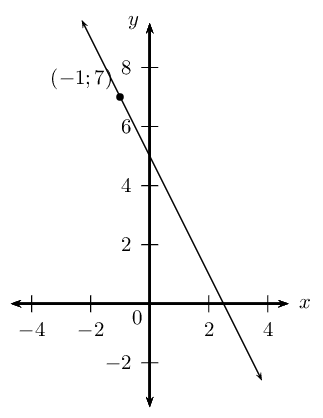### Write down the gradient–intercept form of straight line equation

$y =mx + c$

Substitute the value of the gradient

$y = -2x + c$

Substitute the coordinates of the given point and find $$c$$

\begin{align*} y &= -2x + c \\ 7 &= -2(-1) + c \\ 7 - 2 &= c \\ \therefore c &= 5 \end{align*}

This gives the $$y$$-intercept $$(0;5)$$.

The equation of the straight line is $$y = -2x + 5$$.

If we are given two points on a straight line, we can also use the gradient–intercept form to determine the equation of a straight line. We solve for the two unknowns $$m$$ and $$c$$ using simultaneous equations — using the methods of substitution or elimination.

## Worked example 7: The gradient–intercept form of straight line equation

Determine the equation of the straight line passing through the points $$(-2;-7)$$ and $$(3;8)$$.

### Draw a sketch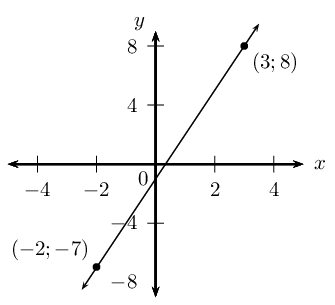### Write down the gradient–intercept form of straight line equation

$y =mx + c$

### Substitute the coordinates of the given points

$\begin{array}{rll} -7 & = m(-2) + c & \\ -7 & = -2m + c & \ldots (1) \\ \\ 8 & = m(3) + c & \\ 8 & = 3m + c & \ldots (2) \end{array}$

We have two equations with two unknowns; we can therefore solve using simultaneous equations.

### Make the coefficient of one of the variables the same in both equations

We notice that the coefficient of $$c$$ in both equations is $$\text{1}$$, therefore we can subtract one equation from the other to eliminate $$c$$:

\begin{align*} -7 &= -2m + c \\ -( 8 &= 3m + c ) \\ -15 &= -5m \\ \therefore 3 &= m \end{align*}

Substitute $$m=3$$ into either of the two equations and determine $$c$$: \begin{align*} -7 &= -2m + c \\ -7 &= -2(3) + c \\ \therefore c &= -1 \end{align*} or \begin{align*} 8 &= 3m + c \\ 8 &= 3(3) + c \\ \therefore c &= -1 \end{align*}

The equation of the straight line is $$y = 3x - 1$$.

## The gradient–intercept form of a straight line equation

Textbook Exercise 4.4

Determine the equation of the straight line:

passing through the point $$(\frac{1}{2};4)$$ and with $$m=2$$.

\begin{align*} y &= mx + c \\ y &= 2x + c \\ 4 &= 2\left( \frac{1}{2} \right) + c \\ 4 &= 1 + c \\ \therefore c &= 3 \\ \therefore y &= 2x + 3 \end{align*}

passing through the points $$(\frac{1}{2};-2)$$ and $$(2;4)$$.

\begin{align*} y &= mx + c \\ -2 &= m\left( \frac{1}{2} \right) + c \\ \text{Multiply by } 2: \quad -4 &= m + 2c \ldots (1)\\ 4 &= 2m + c \\ \text{Multiply by } 2: \quad 8 &= 4m + 2c \ldots (2)\\ (2) - (1): \quad 8 + 4 &= 4m - m \\ 12 &= 3m \\ \therefore 4 &= m \\ \therefore c &= 4 - 2(4) \\ &= -4 \\ \therefore y &= 4x - 4 \end{align*}

passing through the points $$(2;-3)$$ and $$(-1;0)$$.

\begin{align*} y &= mx + c \\ -3 &= 2m + c \ldots (1) \\ 0 &= -m + c \ldots (2) \\ (1) - (2): \quad -3 &= 2m + m \\ -3 &= 3m \\ \therefore -1 &= m \\ \therefore c &= -1 \\ \therefore y &= -x - 1 \end{align*}

passing through the point $$(2;-\frac{6}{7})$$ and with $$m=-\frac{3}{7}$$.

\begin{align*} y &= mx + c \\ y &= -\frac{3}{7}x + c \\ -\frac{6}{7} &= -\frac{3}{7}(2) + c \\ -\frac{6}{7} + \frac{6}{7} &= + c \\ \therefore c &= 0 \\ \therefore y &= -\frac{3}{7}x \end{align*}

which cuts the $$y$$-axis at $$y=-\frac{1}{5}$$ and with $$m=\frac{1}{2}$$.

\begin{align*} y &= mx + c \\ y &= mx - \frac{1}{5} \\ \therefore y &= \frac{1}{2}x -\frac{1}{5} \end{align*}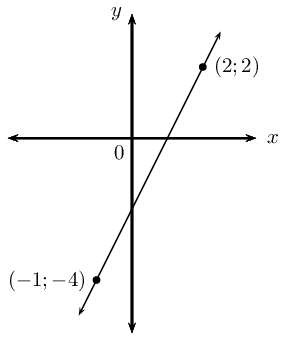\begin{align*} y &= mx + c \\ 2 &= 2m + c \ldots (1) \\ -4 &= -m + c \ldots (2) \\ (1)-(2) \quad 2 + 4 &= 2m + m \\ 6 &= 3m \\ \therefore m &= 2 \\ \therefore c &= -4 +2 \\ &= -2\\ \therefore y &= 2x -2 \end{align*}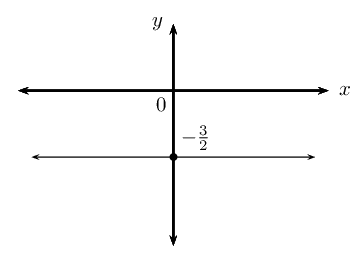$$y = -\frac{3}{2}$$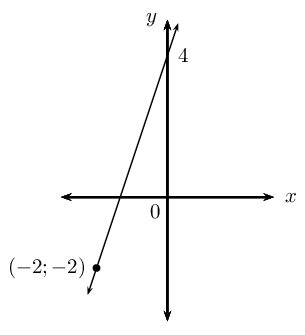\begin{align*} c &= 4 \\ y &= mx + c \\ y &= mx + 4 \\ \text{Subst. } (-2;-2) \quad -2 &= -2m + 4 \\ -2m &= -6 \\ m &= 3 \\ \therefore y &= 3x + 4 \end{align*}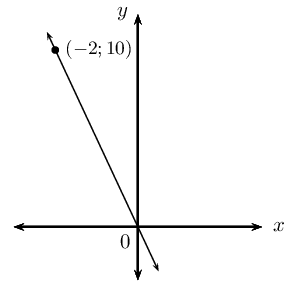\begin{align*} c &= 0 \\ y &= mx + c \\ y &= mx + 0\\ \text{Subst. } (-2;10) \quad 10 &= -2m \\ -2m &= 10 \\ \therefore m &= -5 \\ \therefore y &= -5x \end{align*}